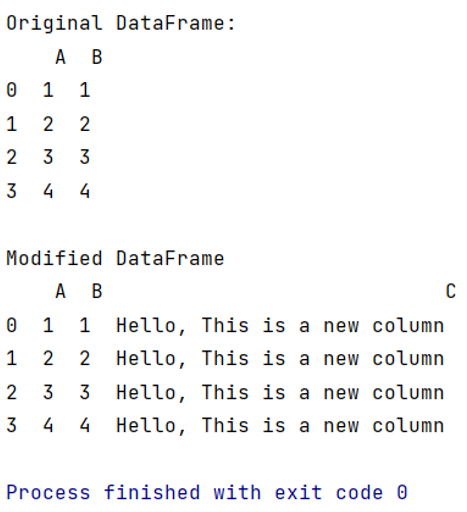# How to add a column to DataFrame with constant value?

Given a DataFrame, we have to add a column to DataFrame with constant value. By Pranit Sharma Last updated : September 20, 2023

Columns are the different fields which contains their particular values when we create a DataFrame. We can perform certain operations on both rows & column values. Sometimes we need to add a column in our dataset and this creation depends upon some condition.

## Problem statement

Here, we are creating a new column in the DataFrame where all the values of the column are same i.e., constant.

## Adding a column to DataFrame with constant value

For this purpose, we will use pandas.Series() method inside it, we will assign the constant value to the columns.

Let us understand with the help of an example:

## Python program to add a column to DataFrame with constant value

```# Importing Pandas package as pd
import pandas as pd

# Creating a dictionary
d = {
'A':[1,2,3,4],
'B':[1,2,3,4]
}

# Creating DataFrame
df = pd.DataFrame(d)

# Display original DataFrame
print("Original DataFrame:\n",df,"\n")

# Creating a new column with similar/ constant value
df['C'] = pd.Series(['Hello, This is a new column' for i in range(len(df.index))])

# Display modified DataFrame
print("Modified DataFrame\n",df)
```

### Output

The output of the above program is: